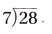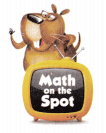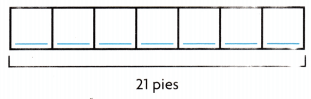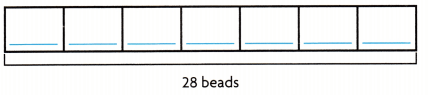# Texas Go Math Grade 3 Lesson 13.2 Answer Key Divide by 7

Refer to our Texas Go Math Grade 3 Answer Key Pdf to score good marks in the exams. Test yourself by practicing the problems from Texas Go Math Grade 3 Lesson 13.2 Answer Key Divide by 7.

## Texas Go Math Grade 3 Lesson 13.2 Answer Key Divide by 7

Essential Question
What strategies can you use to divide by 7?

Unlock the Problem
Erin used 28 large apples to make 7 apple pies. She used the same number of apples for each pie. How many apples did Erin use for each pie?

• Do you need to find the number of equal groups or the number in each group?

One Way Make an array.

• Draw 1 tile in each of 7 rows.
• Continue drawing 1 tile in each of the 7 rows until all 28 tiles are drawn.
• Count the number of tiles in each row.
• There are ___ tiles in each row.

So, Erin used ___ for each pie.
You can write 28 ÷ 7 = __ orMath Talk
Mathematical processes
Why can you use division to solve the problem? Explain.

Other Ways
A. Use a related multiplication fact.B. Make equal groups.

• Draw 7 circles to show 7 groups.
• Draw 1 counter in each group.
• Continue drawing 1 counter at a time until all 28 counters are drawn.

There are __ counters in each group.

Share and Show

Question 1.
Use the related multiplication fact to find 42 ÷ 7. ______________
6 × 7 = 42
42 ÷ 7 = ___

Math Talk
Mathematical Processes
Explain why you can use a related multiplication fact to solve a division problem.

Find the unknown factor and quotient.

Question 2.
7 × ___ = 7 7 ÷ 7 = ___

Question 3.
7 × ___ = 35 35 ÷ 7 = ___

Find the quotient.

Question 4.
__ = 14 ÷ 7

Question 5.
48 ÷ 6 = ___

Question 6.
0 ÷ 7 = ___

Question 7.
___ = 18 ÷ 2

Unlock the Problem

Question 8.
H.O.T. Gavin sold 21 pies to 7 different people. Each person bought the same number of pies. How many pies did Gavin sell to each person?(A) 3
(B) 4
(C) 14
(D) 28
a. What do you need to find?

b. How can you use a strip diagram to help you decide which operation to use to solve the problem?

c. Complete the strip diagram to help you find the number of pies Gavin sold to each person.d. What is another way you could have solved the problem?

e. Write a related multiplication fact.

f. complete the sentences.
Gavin sold ___ pies to ___ different people.
Each person bought the same number of ___.
So, Gavin sold __ pies to each person.

g. Fill in the bubble for the correct answer choice above.

Question 9.
H.O.T. Multi-Step There are 35 peach pies and 42 cherry pies on 7 shelves at the bakery. It each shelf has the same number of peach pies and apple pies, how many pies are on each shelf? Explain.

Fill in the bubble for the correct answer choice. You may use a division strategy to help you solve.

Question 10.
Lee took his dog on hikes 49 days last year. There are 7 days in every week. How many weeks of the year did Lee and his dog hike?
(A) 6
(B) 7
(C) 5
(D) 10

Question 11.
At the Twin Maples neighborhood carnival, 63 people play games. They play in groups of 7 people. How many groups are there?
(A) 7
(B) 6
(C) 8
(D) 9

Question 12.
Multi-Step There are 31 girls and 25 boys in the marching band. When the band marches, they are in 7 rows. How many people are in each row?
(A) 8
(B) 7
(C) 9
(D) 10

Texas Test Prep

Question 13.
Clare bought 28 peaches to make pies for the bake sale. She used 7 peaches for each pie. How many pies did Clare make?
(A) 6
(B) 4
(C) 3
(D) 5

### Texas Go Math Grade 3 Lesson 13.2 Homework and Practice Answer Key

Find the unknown factor and quotient.

Question 1.
7 × __ = 21
21 ÷ 7 = ___

Question 2.
7 × __ = 56
56 ÷ 7 = ___

Find the unknown number.

Question 3.
63 ÷ 7 = ___

Question 4.
7 = 42 ÷ ___

Question 5.
49 ÷ __ = 7

Question 6.
70 ÷ __ = 10

Question 7.
28 ÷ 7 = ___

Question 8.
__ = 21 ÷ 7

Problem Solving

Question 9.
Regina has 28 beads. She puts them into 7 equal groups to make a design. How many beads are in each group?
Complete the bar model to solve the problem.There are __ beads in each group.

Question 10.
What is a related multiplication fact that could solve the problem?

Question 11.
Mick has 56 counters. He can divide 56 into 7 equal groups. How else can Mick break apart the 56 counters into equal groups of 7?

Texas Test Prep

Question 12.
Nyala has 63 paper flowers. She makes 7 bunches of flowers as centerpieces. Each centerpiece has the same number of flowers. How many flowers are in each centerpiece?
(A) 7
(B) 8
(C) 6
(D) 9

Question 13.
A group of 42 students are at a puppet show. They sit in equal rows of 7. In how many rows are the students sitting?
(A) 7
(B) 6
(C) 49
(D) 35

Question 14.
A chef uses 49 pounds of beef to make 7 pots of chili. If each pot has the same amount of beef, how many pounds of beef are in each pot?
(A) 8 pounds
(B) 6 pounds
(C) 7 pounds
(D) 9 pounds

Question 15.
Hank uses 35 potatoes to make 7 bowls of potato salad Each bowl has an equal number of potatoes. How many potatoes are in each bowl?
(A) 6
(B) 5
(C) 7
(D) 4

Question 16.
Multi-Step Ed has 42 red beads and 28 blue beads. He uses an equal number of all the beads to decorate 7 clay sculptures. How many beads are on each sculpture?
(A) 3
(B) 10
(C) 4
(D) 8